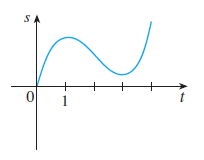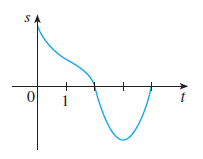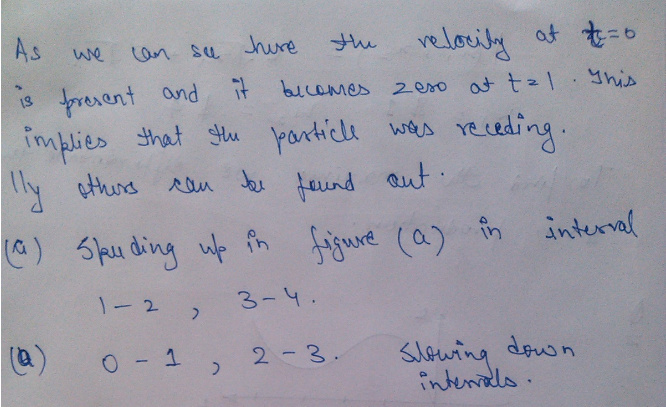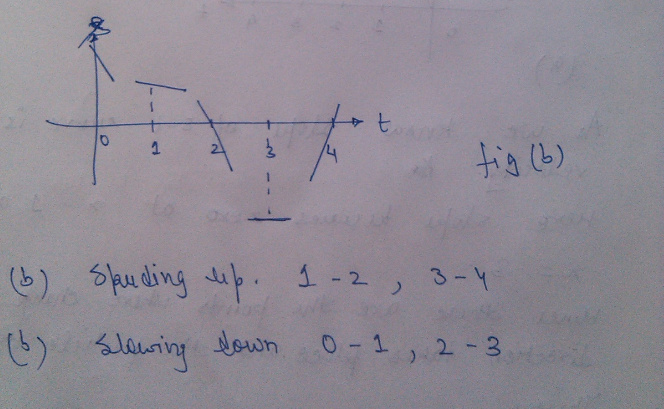# When is the particle speeding up? When is it slowing down?

When is the particle speeding up? When is it slowing down?

Graphs of the position functions of two particles are shown, where t is measured in seconds.
(a)When is the particle in figure (a) speeding up? (Enter your answer using interval notation.)

(b)When is the particle in figure (b) speeding up? (Enter your answer using interval notation.)